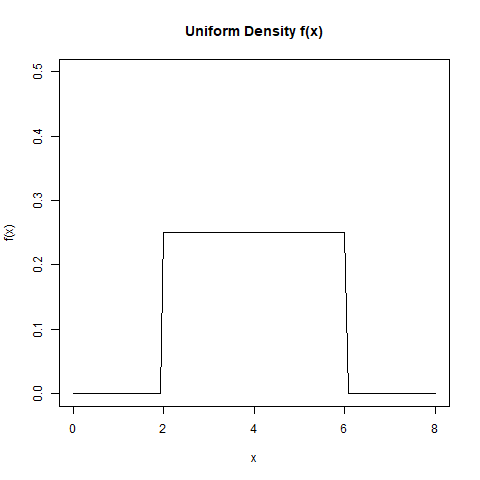# Compute Density of the Distribution Function in R Programming – dunif() Function

`dunif()` function in R Language is used to provide the density of the distribution function.

Syntax:
dunif(x, min = 0, max = 1, log = FALSE)

Parameters:
x: represents vector
min, max: represents lower and upper limits of the distribution
log: represents logical value for probabilities

Example 1:

 `# Create vector of random deviation ` `u <- ``runif``(20) ` ` `  `dunif``(u) == u ` ` `  `print``(``dunif``(u)) `

Output:

```
 FALSE FALSE FALSE FALSE FALSE FALSE FALSE FALSE FALSE FALSE FALSE FALSE
 FALSE FALSE FALSE FALSE FALSE FALSE FALSE FALSE
 1 1 1 1 1 1 1 1 1 1 1 1 1 1 1 1 1 1 1 1
```

Example 2:

 `# Output to be present as PNG file ` `png``(file = ``"dunifGFG.png"``) ` ` `  `# Plot density ` `curve``(``dunif``(x, min = 2, max = 6), 0, 8, ylim = ``c``(0, 0.5), ` `      ``ylab = ``"f(x)"``, main = ``"Uniform Density f(x)"``) ` ` `  `# Saving the file ` `dev.off``() `

Output:My Personal Notes arrow_drop_upCheck out this Author's contributed articles.

If you like GeeksforGeeks and would like to contribute, you can also write an article using contribute.geeksforgeeks.org or mail your article to contribute@geeksforgeeks.org. See your article appearing on the GeeksforGeeks main page and help other Geeks.

Please Improve this article if you find anything incorrect by clicking on the "Improve Article" button below.

Article Tags :

Be the First to upvote.

Please write to us at contribute@geeksforgeeks.org to report any issue with the above content.